##### Actions

(diff) ← Older revision | Latest revision (diff) | Newer revision → (diff)

The field concerned with best rational approximation to power series. Let(1)

be an arbitrary power series (formal or convergent), and let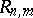() be the class of all rational functions of typewhereandare polynomials in,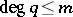,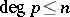and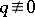. A Padé approximant of typeto the power series (1) (the function) is a rational function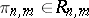having the maximum possible order of contact in the classwith the power series (1) at the point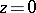. More precisely, the functionis determined by the condition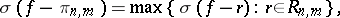whereis the index of the first non-zero coefficient of the series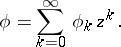It is also possible to determine the function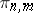as the quotientof arbitrary polynomialsand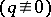satisfying the conditions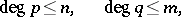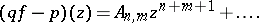(2)

For fixedthere exists a unique Padé approximantto the power series (1). The table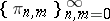is called the Padé table of the series (1). The sequences of typeare called the rows of the Padé table (the zero row coincides with the sequence of Taylor polynomials of);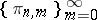are called the columns of the Padé table; and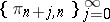are called the diagonals of the Padé table. The most important special caseis the principal diagonal.

The calculation of the functionsreduces to the solution of a system of linear equations whose coefficients are expressed in terms of the coefficients,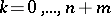, of the given power series. If the Hankel matrix,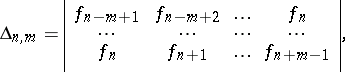has determinant non-zero, then the denominatorof the functionis given by the formula(the normalization is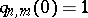; an explicit formula can also be written down for the numerator of the function). Moreover,The latter relation is sometimes taken as the definition of a Padé approximant; in this case a Padé approximant need not exist for certain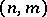. The Padé approximant of typeof the given power seriesis often denoted by the symbolFor an effective calculation of Padé approximants it is more convenient to make use not of explicit formulas but of recurrence relations existing in the Padé table. A large number of algorithms have been constructed for the automatic calculation of a Padé approximant; these problems are of specific importance in connection with applications (see , ).

The first general problem concerning the interpolation of given values of a function at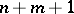different points by means of rational functions of classwas considered by A.L. Cauchy ; C.G.J. Jacobi  extended Cauchy's results to the case of multiple-point interpolation. The case of one-multiple point corresponds to Padé approximation. The idea of Padé approximation was formulated at the end of the 19th century within the classical theory of continued fractions (G. Frobenius , H. Padé ). Fundamental results on diagonal Padé approximants were obtained by P.L. Chebyshev, A.A. Markov and T.J. Stieltjes in terms of continued fractions. They discovered and studied the relations of diagonal Padé approximants with orthogonal polynomials, quadrature formulas, moment problems, and other problems of classical analysis (see –). The origin of the study of the rows of the Padé table lays in the work on the radius of meromorphy of a function defined by a power series and on the convergence of the row of the Padé table in the discs of meromorphy (see , ).

From the beginning of the 20th century onwards Padé approximation has become an independent object of analysis and constitutes an important chapter in the theory of rational approximation of analytic functions. Using local data (coefficients of a power series) for their construction, they allow one to study global properties of the corresponding analytic function (analytic continuation, the character and distribution of singularities, etc.) and to compute the value of a function outside the disc of convergence of the power series.

Along with classical Padé approximation, various generalizations have been considered: general interpolation processes by means of rational functions with free poles (multiple-point Padé approximation); rational approximation of series with respect to given systems of polynomials (e.g. with respect to orthogonal polynomials); joint Padé approximation (Padé–Hermite approximation); rational (Padé type) approximation of power series of several variables, and other topics.

How to Cite This Entry: